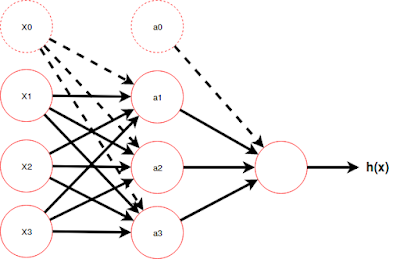## Thursday, October 26, 2017

### 3. Notes on Machine Learning: Basics of Neural NetworksNeural Networks is an interesting branch in machine learning which attempts to mimic the functionality of neurons in human brain. A neural network consists of the input feature vector $$X$$ to a node, the hypothesis function which is sometimes called the activation function running inside a node and finally the output of the function. Instead of having a single activation unit, we can have multiple layers of activation nodes. The input vector layer is considered as the first layer while there are multiple hidden layers (layer 2, layer 3, etc) before the output layer.

The $$\theta$$ parameter set is not a single vector like in linear regression and logistic regression in this case. This time, in neural networks, we have a  $$\theta$$ parameter set between every two layers. For example in the above figure, we have three layers, and therefore, we have two $$\theta$$ sets. The arrows going from layer 1 to layer 2 represent the parameter set $$\theta^{(1)}$$. The arrows going from layer 2 to layer 3 represent the parameter set $$\theta^{(2)}$$. The upperscript number within the brackets represent the origin layer this parameter set belongs to. Furthermore, $$\theta^{(1)}$$ is a matrix with 3x4 dimentions. There, every raw represents the set of arriows coming from the layer 1 features to a node in layer 2. For example, the element $$\theta^{(1)}_{10}$$ represents the arrow to $$a_1^{(2)}$$ from $$x_0$$. The element $$\theta^{(1)}_{20}$$ represents the arrow to $$a_2^{(2)}$$ from $$x_0$$.

$$\theta^{(1)} = \begin{bmatrix}\theta^{(1)}_{10} & \theta^{(1)}_{11} & \theta^{(1)}_{12} & \theta^{(1)}_{13}\\\theta^{(1)}_{20} & \theta^{(1)}_{21} & \theta^{(1)}_{22} & \theta^{(1)}_{23}\\\theta^{(1)}_{30} & \theta^{(1)}_{31} & \theta^{(1)}_{32} & \theta^{(1)}_{33}\end{bmatrix}$$

Meanwhile $$\theta^{(2)}$$ is a raw vetor (1x4) in this case. This is because there are 4 arrows coming from the layer 2 nodes to the layer 1 node.

$$\theta^{(2)} = \begin{bmatrix}\theta^{(2)}_{10} & \theta^{(2)}_{11} & \theta^{(2)}_{12} & \theta^{(2)}_{13}\end{bmatrix}$$

The hypothesis function in thse neural networks is a logistic function just like in the logistic regression.
$$h_\theta (x) = \frac{1}{1 + e^{- \theta^T x}}$$
For a neural network like the one shown in the above figure, we can calculate the activation and get the final output inthe following way. There, $$a_1^{(2)}$$ represent the activation node 1 in the layer 2 (hidden layer). Similarly $$a_2^{(2)}$$ represent the activation node 2 in the layer 2 and so on.

$$a_1^{(2)} = g(\theta_{10}^{(1)} x_{0} + \theta_{11}^{(1)} x_{1} + \theta_{12}^{(1)} x_{2} + \theta_{13}^{(1)} x_{3})$$

$$a_2^{(2)} = g(\theta_{20}^{(1)} x_{0} + \theta_{21}^{(1)} x_{1} + \theta_{22}^{(1)} x_{2} + \theta_{23}^{(1)} x_{3})$$

$$a_3^{(2)} = g(\theta_{30}^{(1)} x_{0} + \theta_{31}^{(1)} x_{1} + \theta_{32}^{(1)} x_{2} + \theta_{33}^{(1)} x_{3})$$

$$h_\theta (x) = a_1^{(3)} = g(\theta_{10}^{(2)} a_{0}^{(2)} + \theta_{11}^{(2)} a_{1}^{(2)} + \theta_{12}^{(2)} a_{2}^{(2)} + \theta_{13}^{(2)} a_{3}^{(2)})$$
Since the hypothesis function is a logistic function, the final output we get is a value between 0 and 1. What we do to have a multiclass classifier is, having multiple nodes in the output layer. Then, we get a unique output value from each node in the output layer representing a specific class.

~************~